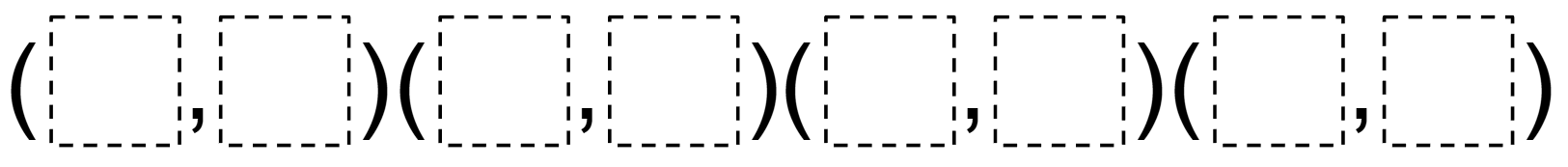# Area of a Quadrilateral on a Coordinate Plane

Directions: Using the digits 0 to 9 at most one time each, fill in the blanks to create a quadrilateral with an area of 16 square units.### Hint

What happens if two ordered pairs have the same x-value?
What happens if two ordered pairs have the same y-value?

One solution is (1,2), (3,0), (5,6), (7,4). Note: If you change the range of integers to be -9 to 9, then the question is also aligned to standard 6.NS.6b.

Source: Daniel Luevanos

## Greatest Common Factor

Directions: Using the digits 0 to 9 at most one time each, place a digit …

1.I am not sure that I agree that this problem is aligned with 6th grade standards. I believe that given the coordinates, 6th graders should be able to compose a larger rectangle by drawing 4 right triangles surrounding the graphed quadrilateral. The resultant rectangle would have coordinates with same first or last coordinates and its area could be found. Students could also find the area of the right triangles and subtract those from the larger rectangle to find the area of the original graphed rectangle. This would correlate with CCSS 6.G.3 I might also change the challenge to allow use of integers between -9 and 9 and allowing them to repeat which would also align with G.1 and G.3 Do you have any other suggestions or is my understanding faulty?
I really like this site and the problems.
Thank you.

•Great suggestion. I can see how this question could be aligned to 6.G.3. If you did change the range of numbers to be -9 to 9, then the question can also be aligned to 6.NS.6b. Glad you like the questions on the website too! This is one of my favorite websites!

•C Farmer, if you are saying it would be very difficult for a 6th grader (pre-Pythagorean Thm) to construct the rectangle to meet the conditions, but that it is reasonable for them to come up with a way to figure out the area for this particular solution, then I agree. Or maybe you’re suggesting giving them the surrounding square and having them carve off right triangles?

2.My sixth grade students successfully reached an answer to this problem today! They are immensely proud of themselves, and I am immensely proud of their perseverance — this problem spanned multiple math periods for us. They propose these coordinates: (3,6), (8,5), (7,2), (1,4). Thank you for challenging my students!

•Daniel Luevanos

This is so awesome, Kimberly! Thank you for sharing! Great work, kids!

3.When we have plotted these points on a Cartesian Plane we have gotten the rectangle, however why does the formula for square units of area not seem to be able to be applied. We have a length of 4 but only a width of 2 units?? Please help!

•I get a 2 unit by 4 unit rectangle, as well, when I plot the ordered pairs for the given answer. This would give an area of 8 square units. Am I missing something?

•I know I am like fives years too late, but if you plot the points of the given answer you will have a rectangle that has been twisted so the length and width are not perpendicular to the horizontal or vertical axis. To find the length and the width you must apply the Pythagorean theorem a^2+b^2 = c^2 when this theorem is applied you will get that the width and the length of the rectangle is √8 and √32 not 2 and 4. √8 x √32= 16

4.I am looking for clarification on this. Are the students expected to know Pythagorean Theorem, to identify the hypotenuse for each triangle? Or are they supposed to draw the rectangular shape and then find the area of each resulting triangle, and subtract from the original area?

5.•What happens if two ordered pairs have the same x-value? The same with y-value

6.What happened to the X and the Y value are both negative numbers

7.1.2 3.4 5.2 3.0

8.9.10.Aylin is a 6th grader at QVMS. I am her father and trying to understand and solve the problem above. We could not figure it out ourselves. But we looked at the proposed solution above by Mrs. Kimberly. We did understand how they did this by using a coordinate system on our board at home. It is a challenging but a great question we think. The way we think Kimberley’s students reach a solution is that they drew a shape of a rectangle on the coordinate system in 16 unit square and rotate it/twisted or manipulated the shape into a shape (keeping the total area fixed) at the coordinate locations that are using numbers 1 thru 9, but not repeating any number. Great exercise. Thank you for the challenge.

11.0,1,2,3,4,5,6,7,8,9

12.just to be are we supposed to post this some where

13.14.1 2 3 0 5 6 7 4

15.16.benjamin leutwyler

1*2 1*2 1*2 1*2

17.(1,2)

18.1,2 3,0 5,6 7,4

19.20.(1.2)(3,0)(5,6)(7,4)

21.(1,2)(3,0)(5,6)(7,4)

22.(1,2) (3,0) (5,6) (7,4)

23.(1,1) (1,5) (4,1) (4,5)

24.(1,2), (3,0), (5,6), (7,4)

25.i think it is 1.2 3.4 5.2 3.0

26.i think its– 1,2 3,0 5,6 7,4

27.I think it’s this 1, 2 3,0 5, 6 7,4

28.I think it is 1, 2 3,0 5, 6 7,4

29.30.I think its 1,2 3,0 5,6 7,4

31.i believe that its 1,2 3,0 5,6 7,4

32.[1,2] [3,0] [5,6] [7,4]

33.(1,2) (3,0) (5,6) (7,4)

34.1,2 3,0 5,6 7,4

35.The answer is(1,2), (3,0) , (5,6) , (7,4)

36.37.01234456.7.8.9